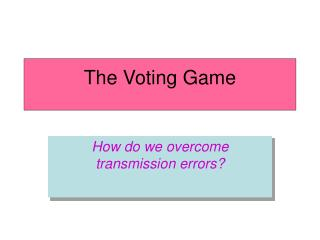DownloadDownload PresentationThe Voting Game

# The Voting Game

Télécharger la présentation## The Voting Game

- - - - - - - - - - - - - - - - - - - - - - - - - - - E N D - - - - - - - - - - - - - - - - - - - - - - - - - - -
##### Presentation Transcript

1. The Voting Game How do we overcome transmission errors?

2. Vote for your favorite animals:

3. Error can occur during the transmission. • In digital transmission, error means: 1  0, or 0  1. • What would happen if one of the “bit” you entered in your vote is affected by transmission error? What can we do to so that our votes won’t be incorrectly registered?

4. Use some math – of course! • What if we add one extra bit to the codes? Now see what happens if one of the bits we entered is affected by transmission error? • Notice the “sum” of all digits in each code is always an even number • This kind of error detection code is called Parity Check.

5. Can parity check code detect any errors? • No. If there are even number of bits affected by the error, the parity check won’t detect it. Cat 0101 0101 0xx1 0011 Butterfly • But having two-bits error is much less likely to than one-bit error. • Parity check can greatly reduce the chance of our votes being incorrectly received. • We can design more complicated codes to detect multi-digit errors, or even correcting the error. But more bits need to be added.

6. Probability Analysis: • If the probability for each bit to be affected by error is 1/10, what is the probability that our vote will not be correctly registered without parity check? 1-(9/10)*(9/10)*(9/10)=0.271 • If the probability for each bit to be affected by error is 1/10, what is the probability that our vote will not be correctly registered with parity check? (two bits error)+(4 bits error)=

7. Without parity check:0.271 = more than 1 out of 4 times our vote will be registered incorrectly. • With parity check:0.0478 = less than 1 out of 20 times our vote will be registered incorrectly. • Chance of error is greatly reduced. • Math did it again!